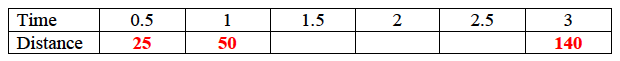### Home > APCALC > Chapter 1 > Lesson 1.1.1 > Problem1-6

1-6.

A car travels $50$ miles per hour for two hours and $40$ miles per hour for one hour. Homework Help ✎

1. Sketch a graph of velocity vs. time. Label the axes with units.

1. Fill out the table below for the distance vs. time.

 Time (hours) $0.5$ $1$ $1.5$ $2$ $2.5$ $3$ Distance (miles)

Consider the Freeway Fatalities problem 1-1... Recall that distance information can be found by calculating the area under a velocity graph.1. Sketch a graph of distance vs. time. Label the axes with units.

Graph the data in the table and connect.
Note that distance is measured in miles and time is measured in hours.